# Demo

Hover on the images to start movies.
Click on an image to start the movie enlarged in a new tab.

### Nucleation and decomposition in a metastable solid solution.

$V/kT = 0.4$, $\overline{c} = 0.9$, $A_n = 0.5$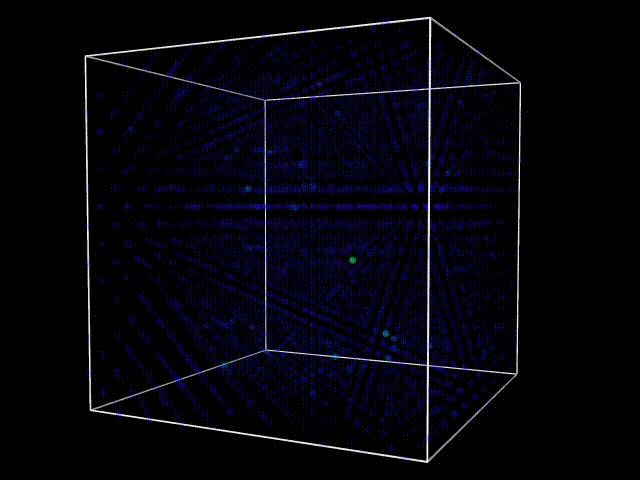A single nucleation process in 20x20x20/2 atoms computation cell.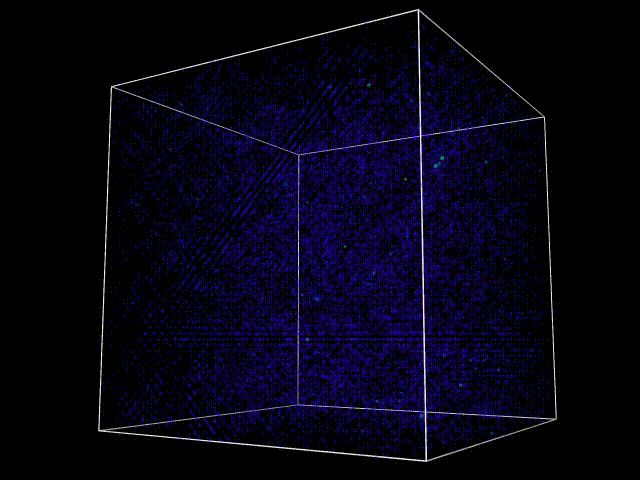Multiple nucleation process in a 60x60x60/2 atoms computation cell

### Influence of noise on the spinodal decomposition in quasi-1D structure (nanowire)

$V/kT = 0.3316$, $\overline{c} = 0.5$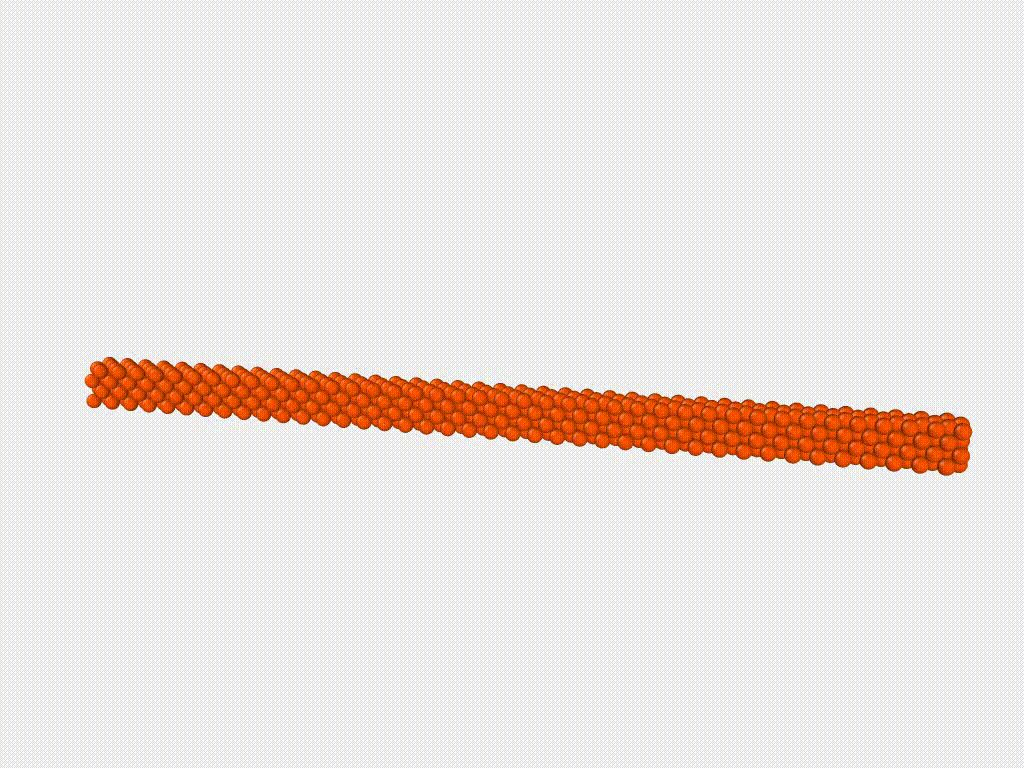Starting from homogeneous state with an initial spike in the middle of the sample to start the decomposition. $(A_n=0)$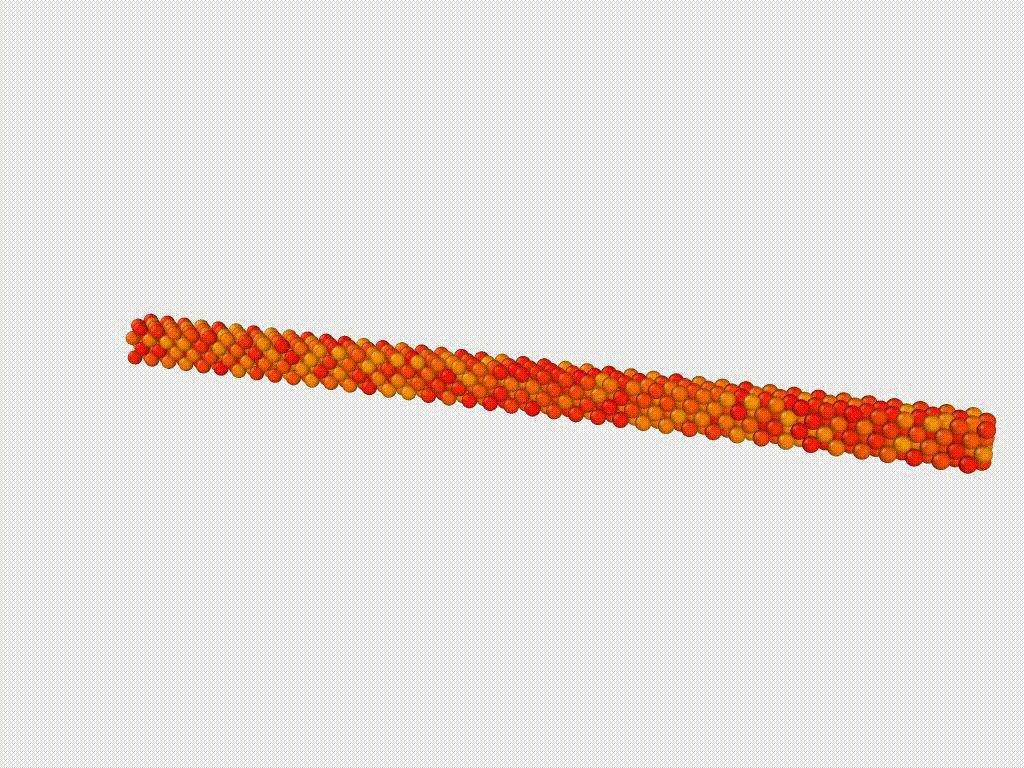Starting from homogeneous state with an initial random noise (amplitude is 0.1, $A_n=0$).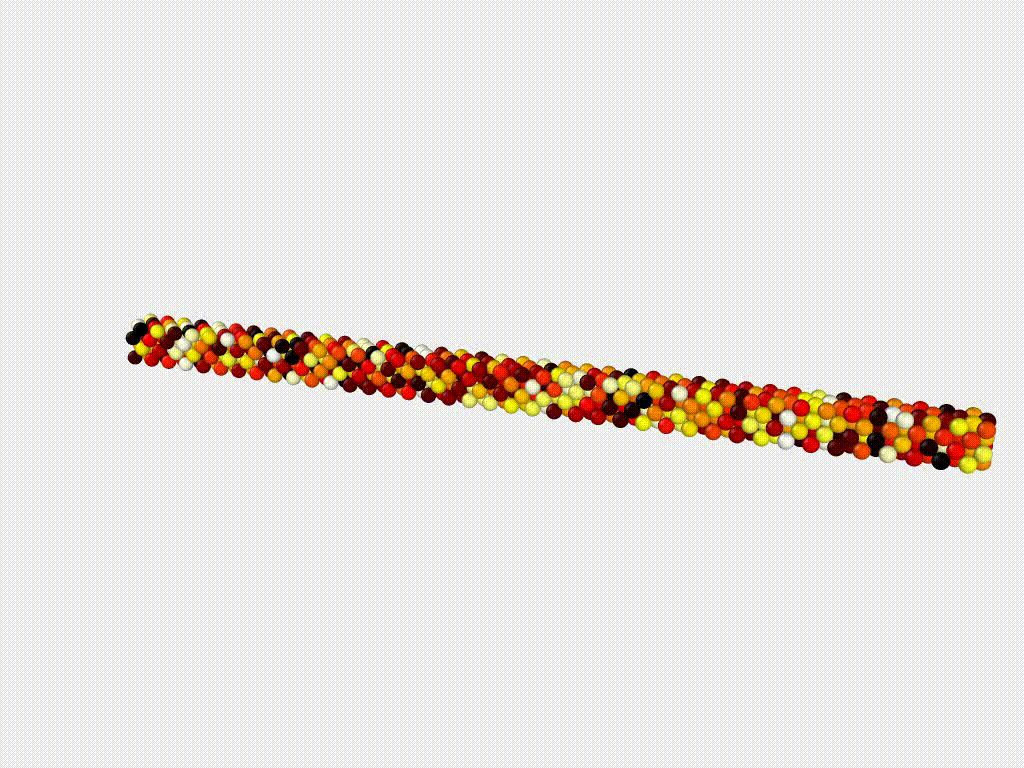Starting from homogeneous state with an initial random noise (amplitude is 0.5, $A_n=0$).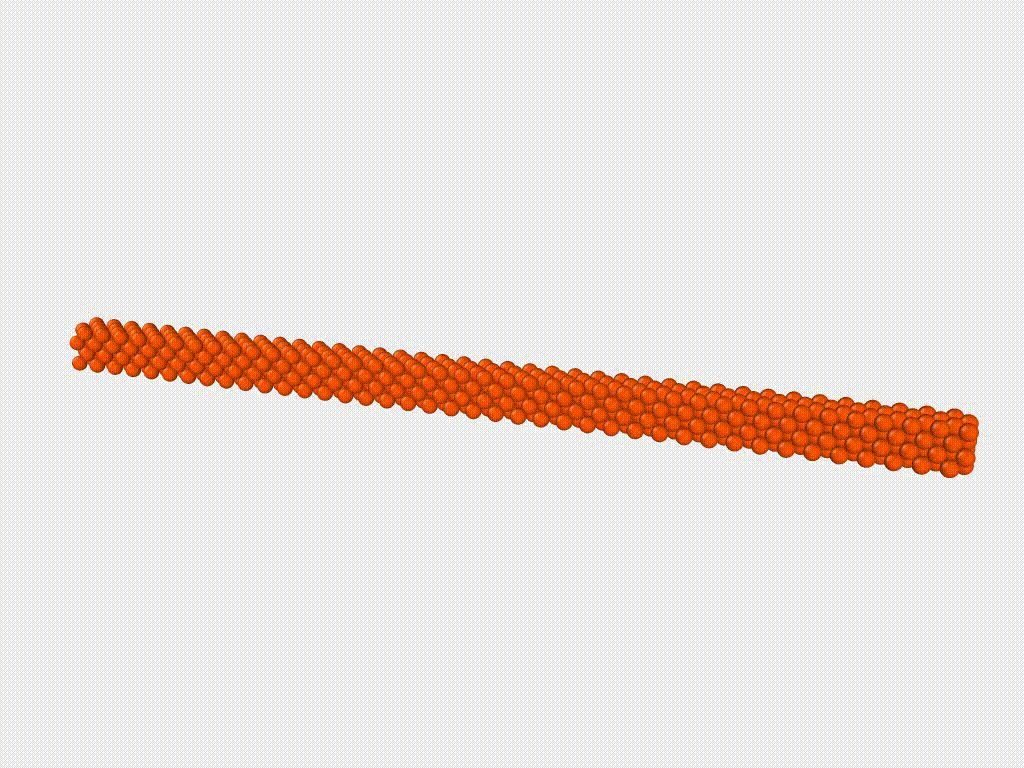Starting from homogeneous state with random (Langevin) noise $A_n = 0.1$.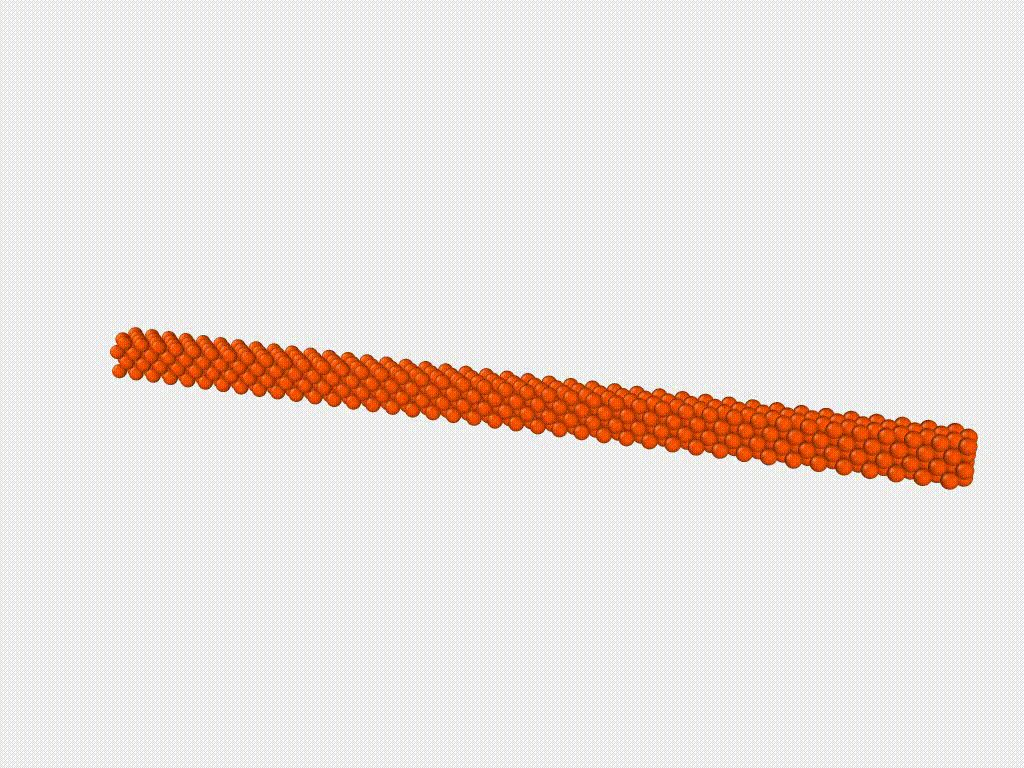Starting from homogeneous state with random (Langevin) noise $A_n = 0.5$.

### Spinodal decomposition in a cubic cell

$V/kT = 0.3316$, $\overline{c} = 0.5$Starting from homogeneous state with spike in the center of the cell $A_n = 0$.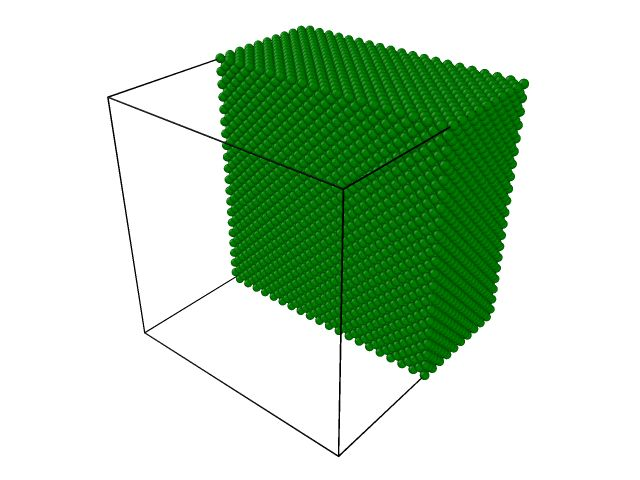Starting from homogeneous state with spike in the center of the cell $A_n = 0$. (half of the cell is shown)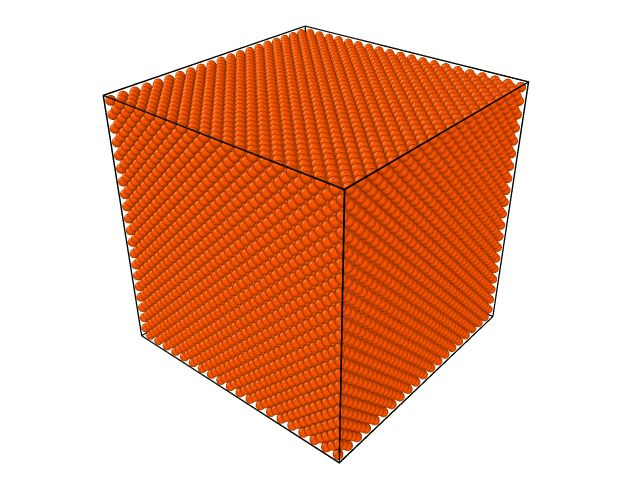Starting from homogeneous state with $A_n = 0.01$. (begging of the process)Starting from homogeneous state with $A_n = 0.01$. (longer and accelerated)

### Ordering in quasi-1D structure (nanowire)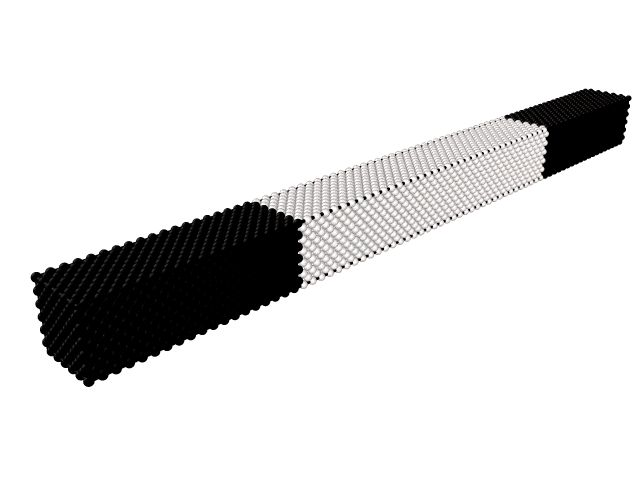Color is proportional to the composition.

### Interface sharpening

(about interface sharpening: SCIENCE, PRL, web)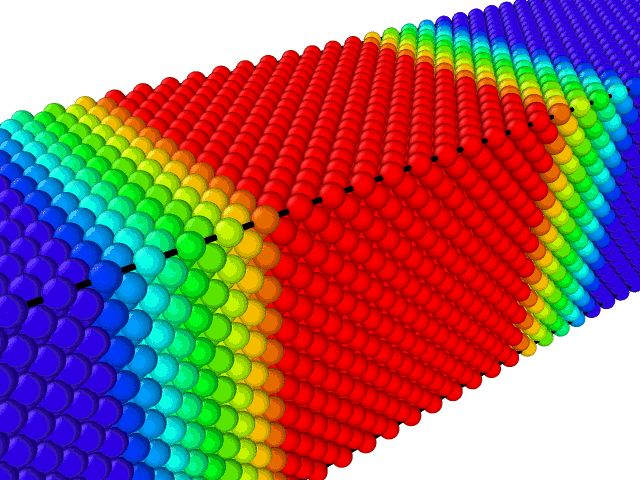Color is proportional to the composition.

Visualization of the results by OVITO (http://www.ovito.org).

A. Stukowski,  Visualization and analysis of atomistic simulation data with OVITO - the Open Visualization ToolModelling Simul. Mater. Sci. Eng. 18 (2010), 015012